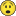# QlikView Scripting

Discussion Board for collaboration on QlikView Scripting.

Announcements

Contributor II

## last 6 months - but consider EOM only

Hi All,

Thanks for your time,

I have the data from April through October but I need to restrict the line chart only for the last six months (do not consider October as it is not yet completed) consider as of last i.e sep EOM only - I have some data for October but not full so need to ignore October

my dimensions are

Cost_Year_Month

Department

expressions is

({<Cost_Year_Month ={">=\$(=Date(addmonths(Max(Cost_Year_Month), -5), 'MMM-YY')) <=\$(=Date(addmonths(Max(Cost_Year_Month), 1),  'MMM-YY'))"} >} Total_Cost

but this is giving me October also - I need to do a max - 1

--------------------------------------------------------------------------------------------------------------------------------------

** Another Question: for another straight table: I have to use a straight table but only show last 6 months

If I have Cost_Year_Month as a dimension along with some other dimensions and say expressions is sum(cost)

is there any easy way of displaying only the last 6 months with these calcs

Thanks for your time

Raj T

4 RepliesNot applicable

## Re: last 6 months - but consider EOM only

Try the following:

({<Cost_Year_Month ={">=\$(=Date(addmonths(Max(Cost_Year_Month)-1, -5), 'MMM-YY')) <=\$(=Date(addmonths(Max(Cost_Year_Month)-1, 1),  'MMM-YY'))"} >} Total_Cost)

-- This should work in a straight table as well.

Honored Contributor III

## Re: last 6 months - but consider EOM only

Hi,

I suggest use your expression in straight table without changing label, it will show the result of your set expression in the header.

I am not sure why didn't you use -1 in 2nd condition when you don't want to use current month data -

<=\$(=Date(addmonths(Max(Cost_Year_Month), 1),  'MMM-YY'))"} >}

Also check if date is properly formatted as I suggested above through straight table expression headerMVP

## Re: last 6 months - but consider EOM only

This?

{<Cost_Year_Month ={">=\$(=Date(MonthStart(Max(Cost_Year_Month), -5), 'MMM-YY')) <=\$(=Date(MonthStart(Max(Cost_Year_Month)) - 1,  'MMM-YY'))"}>}

Logic will get you from a to b. Imagination will take you everywhere. - A Einstein
Contributor II

## Re: last 6 months - but consider EOM only

Thank you Jonathan (Thank you All),

Actually, I got wrong instructions - user says now he wants to see the October also - i.e last 6 months including the October -

(fabs(Sum({<Cost_Year_Month ={">=\$(=Date(addmonths(Max(Cost_Year_Month), -5), 'MMM-YY')) <=\$(=Date(addmonths(Max(Cost_Year_Month), 1),  'MMM-YY'))"} >} Total_Cost)), '\$#,##0')

This expression is giving me the data from May to October in the line chart -  but now I have another issue I have to get the

data points in the line chart to show K, M,B instead of the full number - used something like below with a new expression and

checked values on data points but this is giving me the april data also

if(fabs(sum(Cost_Year_Month))>=1000000,

num(fabs(sum(Cost_Year_Month))/1000000,'#,##0.0 M'),

num(fabs(sum(Cost_Year_Month))/1000,'#,##0 K'))

Thank you all,

RT

Community Browser